# Teaching recurrent Neural Networks about Monet

Recurrent Neural Networks have boomed in popularity over the past months, thanks to articles like the amazing The Unreasonable Effectiveness of Recurrent Neural Networks by Andrej Karpathy.

Long story short, Recurrent Neural Networks (RNNs) are a type of NNs that can work over sequences of vectors and where their elements keep track of their state history.

Neural Networks are increasingly easy to use, specially in the Python ecosystem, with libraries like Caffe, Keras or Lasagne making the assembly of neural networks a trivial task.

I was checking the documentation on Keras and found an example to generate text from Nietzsche readings via a Long Short Term Memory Network (LSTM).

I run the example, and after a couple hours the model started producing pretty convincing, Nietzsche-looking text.Skynet learning about Nietzsche

So I thought in this example the training set is just an array of lines, each line being an array of characters. Images follow a similar data structure

The training dataset consisted of 50 images of Monet Paintings.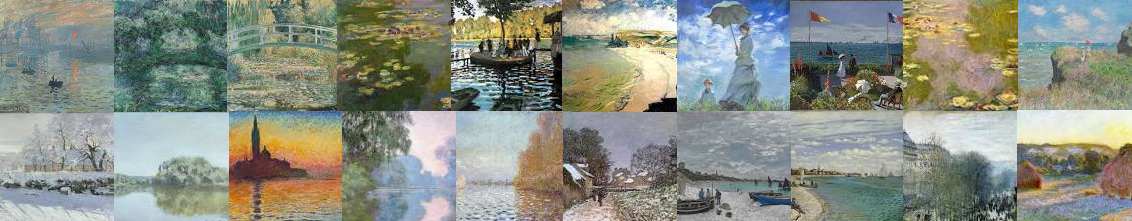Input sample

I loaded the list of images as an array. Each image is initially a width * height * 3 numpy array , with the 3 being 3 numbers containing the Red, Green and Blue mix of the specific pixel.

To convert the 3 dimensional ararys to one dimensional, i used the following trick.

First, I concatenated all the rows on each image into one single row (appending every row after the first one). Then, i converted the RGB values into hex colors, thus converting a length 3 array (r,g,b) to a string (#FFF).

However, the network works only with numerical values, so I took all the colors, put them in a list and then replace every hex color with its position in the list.

So for example, if the color list looks like `[#000, #001, #211, ...,#FFF]`, then a pixel with the rgb values `(0,0,0)` would be converted to the hexcode `#000`, and since that is the first element on the color list, it would be replaced by the array `[1, 0, 0, ....0]`

One issue I found is that, since there were many colors in total, the individual pixel arrays were too big and wouldn't fit in memory. I fixed this by using color quantization. Think of color quantization as a clustering problem that can be solved using K-Means, for example.

Here is the code that loads the images:

``````def rgb_to_hex(array):
array = map(lambda x: int(x * 255), array)
return '{:02x}{:02x}{:02x}'.format(*array)

input_folder = 'monet'
image_width = image_height = 75
input_layer_size = image_width * image_height
input_images = os.listdir(input_folder)
n_samples = len(input_images)
n_colors = 1000 #for color quantization

text = np.empty((0,0))
text = []

print('Reading and Quantizing input images')
for input_image in input_images:
image_data = scipy.misc.imresize(image_data, (image_height, image_width))
image_data = image_data.reshape(input_layer_size,3)
text.append(image_data)
text = np.array(text)
text = quantize(text, n_colors)
import pdb;pdb.set_trace()
text = np.apply_along_axis(rgb_to_hex, 2, text)
text = text.reshape(n_samples*input_layer_size)

chars = np.unique(text)
print('total chars:', len(chars))
char_indices = dict((c, i) for i, c in enumerate(chars))
indices_char = dict((i, c) for i, c in enumerate(chars))
``````

### Network setup

In terms of the Network, i decided to test my theory of the similarities between text and images, and used the same exact implementation that the text example had.

2 stacked LSTM layers with dropout to avoid overfitting and an output layer with softmax activation, and RMSProp as the learning optimizer.

``````model = Sequential()

model.compile(loss='categorical_crossentropy', optimizer='rmsprop')
``````

### Results

LSTM Networks are very interesting, they seem to pick up structures in the data as they learn, with the length of those structures increasing after every iteration.

##### Iterations 1-10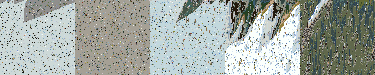Random noise, since the model hasnt pick up any boundaries of hte data yet.

##### Iterations 20-30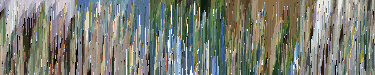By iteration #20, the model its starting to aggregate colors together. However, it hasnt figured out properly the boundaries of these color sets.

##### Iterations 50-60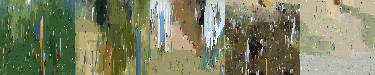At this point the network has pretty much figured out which colors fit well with other colors. It made snowy like paintings, as well as green-nature ones.

##### Iterations 80-100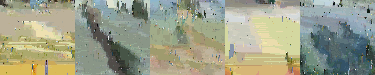Now we are talking! The output was so impressive that i thought the model was overfitting, but the outputs look similar to the input, but not that similar.

The network has gone far enough on the sequences of pixels to understand not only sets of colors that are together, but also the shapes that those sets normally have. The output by iteration #80 is pretty similar in style to Monet paintings.

In conclusion, Recurrent neural networks, given time and a specific input, can generate all kinds of surprising findings.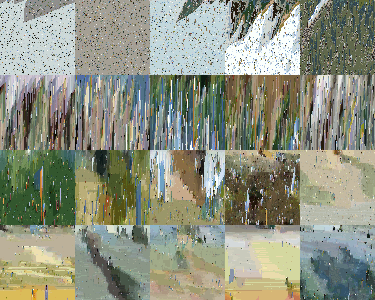Output evolution

Wanna try this at home? You can check it out on github. You will have to install Keras, and i would recommend installing Cuda since this process might be too much for your CPU to handle. (If you are an ubuntu user, you might wanna check this article out)